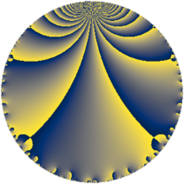# Properties

 Label 30.3.bLevel $30$ Weight $3$ Character orbit 30.b Rep. character $\chi_{30}(29,\cdot)$ Character field $\Q$ Dimension $4$ Newform subspaces $1$ Sturm bound $18$ Trace bound $0$

# Related objects

## Defining parameters

 Level: $$N$$ $$=$$ $$30 = 2 \cdot 3 \cdot 5$$ Weight: $$k$$ $$=$$ $$3$$ Character orbit: $$[\chi]$$ $$=$$ 30.b (of order $$2$$ and degree $$1$$) Character conductor: $$\operatorname{cond}(\chi)$$ $$=$$ $$15$$ Character field: $$\Q$$ Newform subspaces: $$1$$ Sturm bound: $$18$$ Trace bound: $$0$$

## Dimensions

The following table gives the dimensions of various subspaces of $$M_{3}(30, [\chi])$$.

Total New Old
Modular forms 16 4 12
Cusp forms 8 4 4
Eisenstein series 8 0 8

## Trace form

 $$4 q + 8 q^{4} - 4 q^{6} - 32 q^{9} + O(q^{10})$$ $$4 q + 8 q^{4} - 4 q^{6} - 32 q^{9} - 16 q^{10} + 8 q^{15} + 16 q^{16} + 48 q^{19} + 68 q^{21} - 8 q^{24} - 36 q^{25} + 68 q^{30} - 128 q^{31} - 64 q^{34} - 64 q^{36} - 32 q^{40} - 68 q^{45} + 136 q^{46} + 60 q^{49} + 32 q^{51} + 100 q^{54} + 272 q^{55} + 16 q^{60} - 64 q^{61} + 32 q^{64} - 272 q^{66} - 68 q^{69} - 136 q^{70} - 272 q^{75} + 96 q^{76} - 288 q^{79} + 188 q^{81} + 136 q^{84} + 128 q^{85} + 128 q^{90} - 200 q^{94} - 16 q^{96} + 272 q^{99} + O(q^{100})$$

## Decomposition of $$S_{3}^{\mathrm{new}}(30, [\chi])$$ into newform subspaces

Label Dim $A$ Field CM Traces $q$-expansion
$a_{2}$ $a_{3}$ $a_{5}$ $a_{7}$
30.3.b.a $4$ $0.817$ $$\Q(\sqrt{2}, \sqrt{-17})$$ None $$0$$ $$0$$ $$0$$ $$0$$ $$q+\beta _{2}q^{2}+(-\beta _{1}-\beta _{2})q^{3}+2q^{4}+(-2\beta _{2}+\cdots)q^{5}+\cdots$$

## Decomposition of $$S_{3}^{\mathrm{old}}(30, [\chi])$$ into lower level spaces

$$S_{3}^{\mathrm{old}}(30, [\chi]) \cong$$ $$S_{3}^{\mathrm{new}}(15, [\chi])$$$$^{\oplus 2}$$